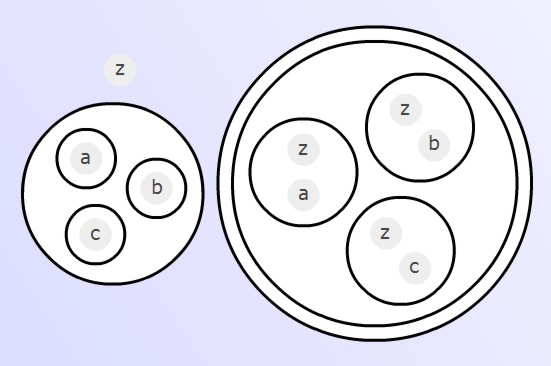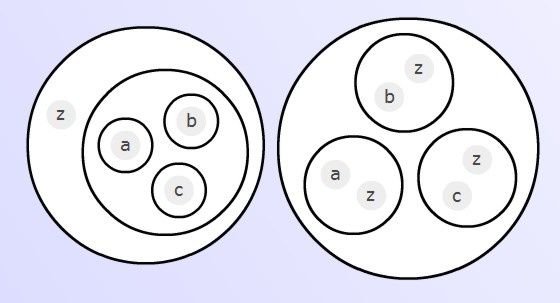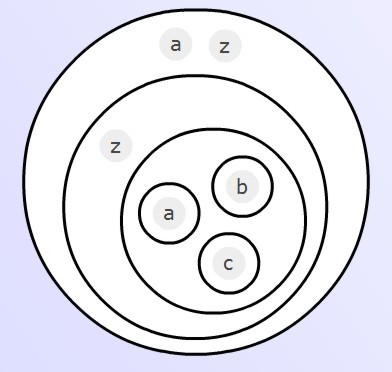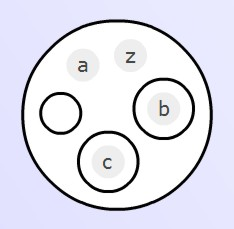### The Mutual Dominance Lemma

The proofs of equivalence, given in the last chapter, have their easy parts and their more difficult parts. In general, the easy parts are where an expression has to be reduced, to _ or O; the difficult parts are where a creative choice has to be made, for example, which expression to copy, where to copy it to, and even worse, what expression to bring into being in the operation we have called undelete. Absolutely any expression may be brought forth out of an empty circle - which sounds marvellous; but really offers too much freedom to the unfortunate calculator.

Reduction is easier than creation: this is what makes the Mutual Dominance Lemma so attractive. As we shall see, it transforms the task of proving an equivalence. Formerly, this was a matter of discovering a sequence of steps, leading from one expression to the other - from A to B, say. Henceforth, there will be an alternative approach, namely, to reduce a third expression, constructed in a standard way out of A and B, to the empty expression.

Given two expressions A and B we say that 'A dominates B', or A≥B, if and only if

AB = A.

Thus A is sufficiently powerful in relation to B that it outshines or eclipses B when put next to it; conversely, if it wants to, it can produce B from under its petticoat.

The second initial equation (delete) tells us that the empty mark O dominates every expression:

O A = O;

on the other hand, it is of the very essence of the empty expression that it is dominated by every expression. It is the peerless doormat among expressions:

A _ = A.

Here are some easy lemmas concerning the relation of dominance:

Lemma 1: Let C and D be any two expressions. Then

CD≥C ;

in other words, a product dominates its factors.

Proof: CDC = CD, by uncopy.

Lemma 2: Suppose A dominates B and B dominates C. Then A dominates C.

Proof: We are given that AB=A and BC=B. Therefore AC=ABC=AB=A.

Lemma 3: If A dominates B then (B) dominates (A).

Proof: Given that AB=A, then (A)(B)=(AB)(B)=(AB(B))(B)=(B).

Lemma 4: A≥B if and only if (A(B))= _ .

Proof: Given that AB=A, then

(A(B)) = (A(AB))   [copy]
= (A(A)) [by the premise]
= _ [complements]

Conversely, given that (A(B))= _ , then

AB = A((B)) = A(A(B)) = A,

so A dominates B.

Lemma 5: Any expression dominates itself.

Proof: AA = A.

Lemma 6: If A≥B and B≥A then A=B.

Proof:

A = AB = B.

Remark: By Lemma 4, we have the following alternative statement of Lemma 6:

If (A(B)) = _ and ((A)B) = _ then A=B.

We will refer to Lemma 6 as the 'Mutual Dominance Lemma'. It allows us to break the task of proving equivalence between A and B into two parts, each partial task consisting of reducing an expression to the empty expression.

Lemma 7: If A dominates B, and if A can be reduced to _ , then so can B.

Proof: _ = A ≥ B; but B ≥ _ (because _ is dominated by all expressions). Therefore B = _ by Lemma 6.

Lemma 8: If (A(B))((A)B) = _ then A=B; and conversely.

The converse is obvious; the main statement can be proved directly, as will be shown below, but it also follows from the Mutual Dominance Lemma with the help of the following Void Factor Theorem.

Void Factor Theorem: If a product AB is known to be equivalent to the void expression, then so must be the factors A and B. Thus AB = _ implies A = _ and B = _ .

Proof:

A = AAB [premise]
= AB [uncopy]
= _   [premise].

(Or: AB≥A by Lemma 1, then apply Lemma 7.) Similarly, B = _ .

Applying this theorem to the case in hand, if the expression given in Lemma 8 is equivalent to _ , then so are both the factors, and thus the premises of Lemma 6 are satisfied.

Direct proof of Lemma 8: given that (A(B))((A)B) = _ , then

A = A (A(B))((A)B) [premise]
= A (A(B))(A(B))((A)B) [copy]
= A ((B))(A(B))((A)B) [uncopy]
= AB(A(B))((A)B) [unwrap]
= ((A))B (A(B))((A)B) [wrap]
= ((A)((A)B))B (A(B))((A)B) [copy]
= ((A)((A) ))B (A(B))((A)B) [uncopy]
= B (A(B))((A)B) [complements]
= B [premise].

Definition: Given expressions A and B, we term the expression

(A(B))((A)B)

the equivalence expression formed out of A and B, and use for it the abbreviation

[A|B].

When we are working graphically, as opposed to typographically, we will use the formThe topic of the equivalence expression is explored in a separate page Development of the Equivalence Expression. For the moment, we just note the important fact that, thanks to Lemma 8, the task of proving two expressions equivalent can always be changed into the task of proving that a third expression - namely, [A|B] - can be reduced to the void _ .

Example: Let us prove the validity of the distribute operation by Mutual Dominance. We use the following variant form of the Lemma:

If A(B) = O and (A)B = O then A = B.

The equation to be proved is

z ( (a)(b)(c)(d)... ) = ( (za) (zb) (zc) (zd) ... ),

so we have to prove, firstly, left dominates right:

z ( (a)(b)(c)(d)... ) (( (za) (zb) (zc) (zd) ... )) = O

and secondly, right dominates left:

(z ( (a)(b)(c)(d)... )) ( (za) (zb) (zc) (zd) ... ) = O

The first of these is easiest. The expression to be reduced is(drawn with just three inner factors, but there could be any number). The right-hand factor can be unwrapped, then each occurrence of z uncopied. Then (a), (b), (c)... may be uncopied from the left-hand factor, eventually leaving it empty. The empty circle can then destroy everything outside it, and we are left with O. To verify the second dominance relation, we have to reduce the expressionOur first move is to copy the left-hand factor into the circle containing z and a. That inner factor becomesNow uncopy the z, unwrap the inner factors, uncopy a out of (a). The factor has turned intoThe empty circle destroys everything within its sphere of influence, and the factor vanishes. We still have the other inner factors, i.e. (bz) and (cz)... to deal with; but they can be got rid of in exactly the same way. Thus the second factor of the whole expression has been turned into O, which destroys the first factor, and the whole expression reduces to O. The second relation of dominance is proved, and hence the equivalence of the left and right expressions in the original equation.

Now enact the proof in the play area!

Exercise: Prove by Mutual Dominance modified distribute, depth-reduction, flip and each way.